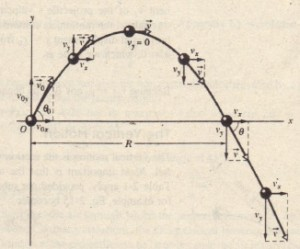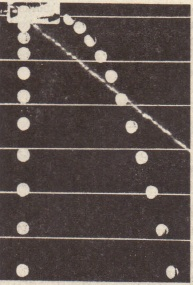# Projectile Motion

Projectile Motion

We next consider a special case of two-dimensional motion. A particle moves in a vertical plane with some initial velocity 170 but its acceleration is always the free fall acceleration g, which is downward. Such a particle is called a projectile (meaning that it is projected or launched) and its motion is called projectile motion. A projectile might be a golf ball  or a baseball in flight, but it is not an airplane or a duck in flight. Our goal here is to analyze projectile motion using the  tools for two-dimensional motion and making the assumption that air has no effect on the projectile.

The components can then be found if we know the angle 80 between V  and the positive x directionProjectile motion like that in, looks complicated, but we have the following simplifying feature (known from experiment).

In projectile motion, the horizontal motion and the vertical motion are independent of  each other, that is, neither motion affects the other. In projectile motion, the horizontal motion and the vertical motion are independent of each other, that is, neither motion affects the other.A stroboscopic photograph ofan orange golf ball bouncing off a hardsurface. Between impacts. the ball hasprojectile motionThe path of a projectile that is launched at and with an initial velocity . The initial velocity and thevelocities at various points along its path are shown. along withtheir components. Note that the horizontal velocity componentremains constant but the vertical velocity component changescontinuously. The rang~ R is the horizontal distance the projectilehas traveled when it returns to its launch height.

Two Golf Balls

Stroboscopic photograph of two golf balls, one simply released and the other shot horizontally by a spring. The golf balls have the same vertical motion, both falling through the same vertical distance in the same interval of time, The fact that one ball is moving horizontally while it is falling has no effect on its vertical motion; that is, the horizontal and vertical motions are independent.

A Great Student Rouser

Shows a demonstration that has enlivened many a physics lecture. It involves a blow gun G, using a ball as a projectile. The target is a can suspended from a magnet M, and the tube of the blow gun is aimed directly at the can. The experiment is arranged so that the magnet releases the can just as the ball leaves the  blow gun.One ball is released from restat the same instant that another ball isshot horizontally to the right. Theirvertical motions are identical